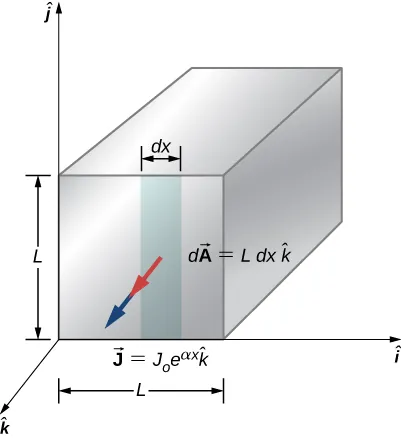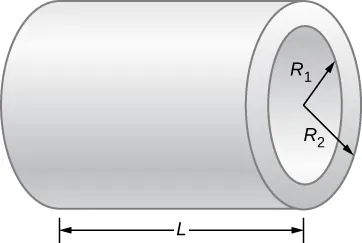University Physics Volume 2

# Challenge Problems

University Physics Volume 2Challenge Problems

### Challenge Problems

81.

A 10-gauge copper wire has a cross-sectional area $A=5.26mm2A=5.26mm2$ and carries a current of $I=5.00AI=5.00A$. The density of copper is $ρ=8.95g/cm3ρ=8.95g/cm3$. One mole of copper atoms $(6.02×1023atoms)(6.02×1023atoms)$ has a mass of approximately 63.50 g. What is the magnitude of the drift velocity of the electrons, assuming that each copper atom contributes one free electron to the current?

82.

The current through a 12-gauge wire is given as $I(t)=(5.00A)sin(2π60Hzt)I(t)=(5.00A)sin(2π60Hzt)$. What is the current density at time 15.00 ms?

83.

A particle accelerator produces a beam with a radius of 1.25 mm with a current of 2.00 mA. Each proton has a kinetic energy of 10.00 MeV. (a) What is the velocity of the protons? (b) What is the number (n) of protons per unit volume? (b) How many electrons pass a cross sectional area each second?

84.

In this chapter, most examples and problems involved direct current (DC). DC circuits have the current flowing in one direction, from positive to negative. When the current was changing, it was changed linearly from $I=−ImaxI=−Imax$ to $I=+ImaxI=+Imax$ and the voltage changed linearly from $V=−VmaxV=−Vmax$ to $V=+VmaxV=+Vmax$, where $Vmax=ImaxRVmax=ImaxR$. Suppose a voltage source is placed in series with a resistor of $R=10ΩR=10Ω$ that supplied a current that alternated as a sine wave, for example, $I(t)=(3.00A)sin(2π4.00st)I(t)=(3.00A)sin(2π4.00st)$. (a) What would a graph of the voltage drop across the resistor V(t) versus time look like? (b) What would a plot of V(t) versus I(t) for one period look like? (Hint: If you are not sure, try plotting V(t) versus I(t) using a spreadsheet.)

85.

A current of $I=25AI=25A$ is drawn from a 100-V battery for 30 seconds. By how much is the chemical energy reduced?

86.

Consider a square rod of material with sides of length $L=3.00cmL=3.00cm$ with a current density of $J→=J0eαxk^=(0.35Am2)e(2.1×10−3m−1)xk^J→=J0eαxk^=(0.35Am2)e(2.1×10−3m−1)xk^$ as shown below. Find the current that passes through the face of the rod.87.

A resistor of an unknown resistance is placed in an insulated container filled with 0.75 kg of water. A voltage source is connected in series with the resistor and a current of 1.2 amps flows through the resistor for 10 minutes. During this time, the temperature of the water is measured and the temperature change during this time is $ΔT=10.00°CΔT=10.00°C$. (a) What is the resistance of the resistor? (b) What is the voltage supplied by the power supply?

88.

The charge that flows through a point in a wire as a function of time is modeled as $q(t)=q0e−t/T=10.0Ce−t/5sq(t)=q0e−t/T=10.0Ce−t/5s$. (a) What is the initial current through the wire at time $t=0.00st=0.00s$? (b) Find the current at time $t=12Tt=12T$. (c) At what time t will the current be reduced by one-half $I=12I0I=12I0$?

89.

Consider a resistor made from a hollow cylinder of carbon as shown below. The inner radius of the cylinder is $Ri=0.20mmRi=0.20mm$ and the outer radius is $R0=0.30mmR0=0.30mm$. The length of the resistor is $L=0.90mmL=0.90mm$. The resistivity of the carbon is $ρ=3.5×10−5Ω·mρ=3.5×10−5Ω·m$. (a) Prove that the resistance perpendicular from the axis is $R=ρ2πLln(R0Ri).R=ρ2πLln(R0Ri).$ (b) What is the resistance?90.

What is the current through a cylindrical wire of radius $R=0.1mmR=0.1mm$ if the current density is $J=J0RrJ=J0Rr$, where $J0=32000Am2?J0=32000Am2?$

91.

A student uses a 100.00-W, 115.00-V radiant heater to heat the student’s dorm room, during the hours between sunset and sunrise, 6:00 p.m. to 7:00 a.m. (a) What current does the heater operate at? (b) How many electrons move through the heater? (c) What is the resistance of the heater? (d) How much heat was added to the dorm room?

92.

A 12-V car battery is used to power a 20.00-W, 12.00-V lamp during the physics club camping trip/star party. The cable to the lamp is 2.00 meters long, 14-gauge copper wire with a charge density of $n=9.50×1028m−3n=9.50×1028m−3$. (a) What is the current draw by the lamp? (b) How long would it take an electron to get from the battery to the lamp?

93.

A physics student uses a 115.00-V immersion heater to heat 400.00 grams (almost two cups) of water for herbal tea. During the two minutes it takes the water to heat, the physics student becomes bored and decides to figure out the resistance of the heater. The student starts with the assumption that the water is initially at the temperature of the room $Ti=25.00°CTi=25.00°C$ and reaches $Tf=100.00°CTf=100.00°C$. The specific heat of the water is $c=4180Jkg∙Kc=4180Jkg∙K$. What is the resistance of the heater?

Order a print copy

As an Amazon Associate we earn from qualifying purchases.